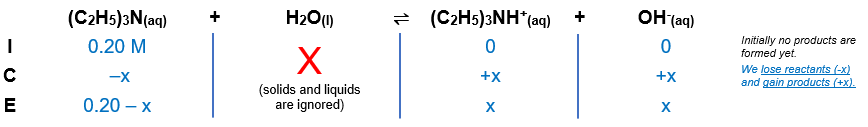# Problem: Calculate [OH-], [H+], and the pH of 0.20 M solution of the following amine.a. triethylamine [(C2H5)3N, Kb = 4.0 X 10 -4]

###### FREE Expert Solution

Equilibrium reaction:        (C2H5)3N(aq) + H2O(l) (C2H5)3NH+(aq) + OH-(aq)

Step 1: Construct an ICE chart for the reaction.Step 2: Write the Kb expression and calculate for Kb.

$\overline{){{\mathbf{K}}}_{{\mathbf{b}}}{\mathbf{=}}\frac{\mathbf{products}}{\mathbf{reactants}}}\phantom{\rule{0ex}{0ex}}{\mathbf{K}}_{\mathbf{b}}\mathbf{=}\frac{\mathbf{\left[}{\mathbf{\left(}{\mathbf{C}}_{\mathbf{2}}{\mathbf{H}}_{\mathbf{5}}\mathbf{\right)}}_{\mathbf{3}}{\mathbf{NH}}^{\mathbf{+}}\mathbf{\right]}\mathbf{\left[}{\mathbf{OH}}^{\mathbf{-}}\mathbf{\right]}}{\left[{\left({C}_{2}{H}_{5}\right)}_{3}N\right]}$

Solids and liquids are not included in the expression

Step 3: Calculate the equilibrium concentrations.

${\mathbf{K}}_{\mathbf{b}}\mathbf{=}\frac{\left[\mathbf{x}\right]\left[\mathbf{x}\right]}{\left[\mathbf{0}\mathbf{.}\mathbf{20}\mathbf{-}\mathbf{x}\right]}\phantom{\rule{0ex}{0ex}}\overline{){{\mathbf{K}}}_{{\mathbf{b}}}{\mathbf{=}}\frac{{\mathbf{x}}^{\mathbf{2}}}{\mathbf{0}\mathbf{.}\mathbf{20}\mathbf{-}\mathbf{x}}}$

$\overline{){\mathbf{x}}{\mathbf{=}}\frac{\mathbf{-}\mathbf{b}\mathbf{±}\sqrt{{\mathbf{b}}^{\mathbf{2}}\mathbf{-}\mathbf{4}\mathbf{a}\mathbf{c}}}{\mathbf{2}\mathbf{a}}}$###### Problem Details

Calculate [OH-], [H+], and the pH of 0.20 M solution of the following amine.

a. triethylamine [(C2H5)3N, Kb = 4.0 X 10 -4]